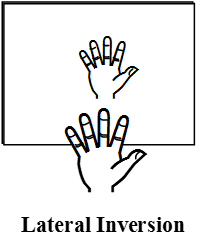# Physics

### Chapter : 3. Light Reflection

#### Deviation

Deviation : δ is defined as the angle between directions of incident ray and emergent ray. So if light is incident at an angle of incidence i,
δ = 180º – (∠i + ∠r) = (180º – 2i) [as ∠i = ∠r]So if light is incident at angle of 30º,
δ = (180º – 2 × 30º) = 120º and for normal incidence ∠i = 0º δ = 180º
Characteristics of the image formed by a plane mirror :
(i) The image formed by a plane mirror is virtual.
(ii) The image formed by a plane mirror is erect.
(iii) The size of the image formed by a plane mirror is same as that of the size of the object. If object is 10 cm high, then the image of this object will also be 10 cm high.
(iv) The image formed by a plane mirror is at the same distance behind the mirror as the object is in front of it. Suppose, an object is placed at 5 cm in front of a plane mirror then its image will be at 5 cm behind the plane mirror.
(v) The image formed by a plane mirror is laterally inverted, i.e., the right side of the object appears as the left side of its image and vice-versa.Lateral Inversion